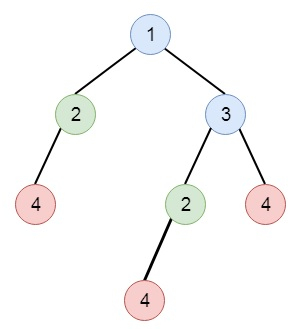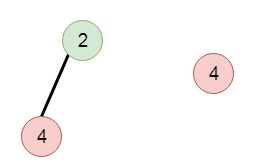# Find Duplicate Subtrees in C++

Suppose we have a binary tree. We have to find all duplicate subtrees. So for each kind of duplicate subtrees, we have to return the root node of any one of them. So suppose we have a tree like −The duplicate subtrees are −To solve this, we will follow these steps −

• Create an array ret, make a map m
• Define a recursive method solve(). This will take node as input. This works as −
• if node is null, then return -1
• x := value of node as string, then concatenate “#” with it.
• left := solve(left of node), right := solve(right of node)
• x := x concatenate “#” concatenate left, concatenate “#” concatenate right
• increase m[x] by 1
• if m[x] is 2, then insert node into ret
• return x
• From the main method call solve(root) and return ret

Let us see the following implementation to get better understanding −

## Example

Live Demo

#include <bits/stdc++.h>
using namespace std;
class TreeNode{
public:
int val;
TreeNode *left, *right;
TreeNode(int data){
val = data;
left = right = NULL;
}
};
void insert(TreeNode **root, int val){
queue<TreeNode*> q;
q.push(*root);
while(q.size()){
TreeNode *temp = q.front();
q.pop();
if(!temp->left){
if(val != NULL)
temp->left = new TreeNode(val);
else
temp->left = new TreeNode(0);
return;
} else {
q.push(temp->left);
}
if(!temp->right){
if(val != NULL)
temp->right = new TreeNode(val);
else
temp->right = new TreeNode(0);
return;
} else {
q.push(temp->right);
}
}
}
TreeNode *make_tree(vector<int> v){
TreeNode *root = new TreeNode(v);
for(int i = 1; i<v.size(); i++){
insert(&root, v[i]);
}
return root;
}
void tree_level_trav(TreeNode*root){
if (root == NULL) return;
cout << "[";
queue<TreeNode *> q;
TreeNode *curr;
q.push(root);
q.push(NULL);
while (q.size() > 1) {
curr = q.front();
q.pop();
if (curr == NULL){
q.push(NULL);
} else {
if(curr->left)
q.push(curr->left);
if(curr->right)
q.push(curr->right);
if(curr->val == 0 || curr == NULL){
cout << "null" << ", ";
} else {
cout << curr->val << ", ";
}
}
}
cout << "]"<<endl;
}
class Solution {
public:
vector <TreeNode*> ret;
map <string, int> m;
string solve(TreeNode* node){
if(!node || node->val == 0){
return "-1";
}
string x = to_string(node->val);
x += "#";
string left = solve(node->left);
string right = solve(node->right);
x = x + "#" + left + "#" + right;
m[x]++;
if(m[x] == 2){
ret.push_back(node);
}
return x;
}
vector<TreeNode*> findDuplicateSubtrees(TreeNode* root) {
ret.clear();
m.clear();
solve(root);
return ret;
}
};
main(){
vector<int> v = {1,2,3,4,NULL,2,4,NULL,NULL,NULL,NULL,4};
Solution ob;
TreeNode *root = make_tree(v);
vector<TreeNode*> trees = ob.findDuplicateSubtrees(root);
for(TreeNode *t : trees){
tree_level_trav(t);
}
}

## Input

[1,2,3,4,null,2,4,null,null,null,null,4]

## Output

[4, ]
[2, 4, ]